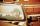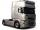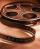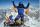Two cars

From the town A to town B started two cars. The first at 7:00 at average speed 60 km per hour, the second at 10:00 at average speed 100 km per hour. The first car will not stay in B, and on the way back meet the second car at half way from A to B. At what time in hours its will meet?

Result

t = 10:45 hSolution:

1.5 s = 60(t-7)
0.5 s = 100(t-10)

1.5s-60t = -420
0.5s-100t = -1000

s = 150
t = 434 = 10.75

Calculated by our linear equations calculator.

Leave us a comment of example and its solution (i.e. if it is still somewhat unclear...):Be the first to comment!To solve this example are needed these knowledge from mathematics:

Do you have a linear equation or system of equations and looking for its solution? Or do you have quadratic equation? Do you want to convert length units?

Next similar examples:

1. TruckIn 7 hours started from town Krnov truck at speed 40 km/h. Passenger car started against it in 8 hours 30 minutes from the city of Jihlava at speed 70 km/h. Distance between this two cities is 225 km. At what time and at what distance from Krnov this two.
2. Warehouse carsFrom the warehouse started truck at speed 40km/h. After 1hour 30mins started from the same place same direction a car at speed 70 km/h. For how long and at what distance from the warehouse overtake a truck?
3. SebastianFrom Kovalov Sebastian walks at 6 km / h started at 8:00 in the direction of Kuty. From Kuty, the godfather is driving at 50 km/h and started at 8:30. The distance is 24 km. When and where grandfather will take Sebastian to the car.
4. Truck PRG-BATruck driving on highway from Prague to Bratislava at average speed of 72 km/h. At the moment when the truck passes 54 kilometer from Prague passenger car departs from Prague which travel to Bratislava at speed 90 km/h. When and where (at waht highway ki
5. MovingVojta left the house at three o'clockat 4 km/h. After half hour later went from the same place Filip by bicycle at speed 18 km/h. How long take Tilip to catch up Vojta and how far from the house?
6. JourneyThe road from A to B measures 11.5 km. Firstly up the hill, then by level plane and then downhill. Tourist goes uphill at 3 km/h, on the plane 4 km/h and downhill 5 km/h. From point A to B went 2h 54 min back 3h 6 min. How long is the segment of level pla
7. Factory and divisionsThe factory consists of three auxiliary divisions total 2,406 employees. The second division has 76 employees less than 1st division and 3rd division has 212 employees more than the 2nd. How many employees has each division?
8. The largerThe larger of two numbers is nine more than four times the smaller number. The sum of the two numbers is fifty-nine. Find the two numbers.
9. Family parcelsIn father will he divided the land so that the older son had three bigger part than younger son. Later elder son gave 2.5 ha field to younger and they had both the same. Determine the area of family parcel.
10. TheatroTheatrical performance was attended by 480 spectators. Women were in the audience 40 more than men and children 60 less than half of adult spectators. How many men, women and children attended a theater performance?
11. Linear systemSolve a set of two equations of two unknowns: 1.5x+1.2y=0.6 0.8x-0.2y=2
12. Three brothersThe three brothers have a total of 42 years. Jan is five years younger than Peter and Peter is 2 years younger than Michael. How many years has each of them?
13. Substitutionsolve equations by substitution: x+y= 11 y=5x-25
14. Ball gameRichard, Denis and Denise together scored 932 goals. Denis scored 4 goals over Denise but Denis scored 24 goals less than Richard. Determine the number of goals for each player.
15. Father and daughterWhen I was 11 my father was 35 year old. Now, father has three times older then me. How old is she?
16. Six yearsIn six years Jan will be twice as old as he was six years ago. How old is he?
17. TreesAlong the road were planted 250 trees of two types. Cherry for 60 CZK apiece and apple 50 CZK apiece. The entire plantation cost 12,800 CZK. How many was cherries and apples?